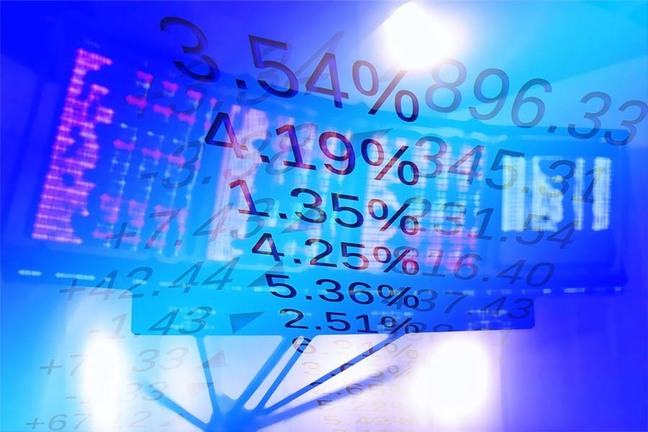## Want to keep learning?

This content is taken from the SOAS University of London's online course, Risk Management in the Global Economy. Join the course to learn more.
4.5

## SOAS University of London# VaR: an application

In this step we will calculate and interpret Value at Risk for a single asset, shares in the BT Group (the UK telecommunications company).

In Week 3 we considered a strategy of investing in BT stock. What is the VaR for this investment position?

We will consider the VaR for one share, but to put the figures into context, consider that one of the largest institutional investors in BT, Invesco Ltd, holds approximately 2.6 million shares in the BT group (www.nasdaq.com).

The formula to calculate VaR is

$VaR = \sigma N^{-1} (X)$

where

$$\sigma$$ is the standard deviation of daily returns on the asset, and

$$N^{-1} (X)$$ is the inverse cumulative standard normal distribution.

The VaR measure is an answer to the question:

What is the maximum loss over a given time period such that there is a low probability … that the actual loss over the given period will be larger? (Crouhy et al, 2001)

We usually choose a probability of 5% or 1%. Using the cumulative standard normal distribution we know there is a probability of 1% of observing a value less than –2.33, and there is a probability of 5% of observing a value less than −1.64.

In Week 3 we estimated that the standard deviation of daily returns on BT stock is 1.9%.

We now have the two elements required to calculate VaR.

The one-day VaR for a 95% confidence level is

$$VaR = 1.9$$% $$\times$$ $$(-1.64)$$ = $$-3.12$$%

There is a 5% chance that the return on the BT stock will be less than –3.12%. Or, put another way, out of 100 trading days, we might expect to see a daily return worse than –3.12% on five days. How does this translate into a value at risk? We also considered an investment in BT stock when the price was £2.24 per share. If the BT stock price was £2.24, a daily return of –3.12% would mean the stock price fell over one day to

$$£2.24$$ $$\times$$ $$e^{-0.0312}$$ = $$£2.17$$

or a fall of £0.07 per share.

To check this, the one-day logged return on that day would be

$1n (2.17) - 1n (2.24) = -0.0312$

The one-day VaR for a 99% confidence level is

$$VaR = 1.9$$% $$\times$$ $$(-2.33)$$ = $$-4.43$$%

So we would expect to see a daily return worse than –4.43% on the BT stock on one day out of 100 trading days. The 99% VaR corresponds to a worse outcome, in the sense of being less likely to occur than the 95% VaR, and the calculated value at risk is correspondingly greater. A daily return of –4.43% corresponds to a fall in the share price to

$$£2.24$$ $$\times$$ $$e^{-0.0443}$$ = $$£2.14$$

or a drop of £0.10 per share.

What is the ten-day VaR on BT stock? The relation between the one-day VaR and the T-day VaR is

$VaR (T\ \text {days}) = VaR (1\ \text {day}) \times \sqrt{T}$

So the ten-day VaR on BT stock for a 95% confidence level is

$$VaR (10\ \text {days}) = -3.12$$% $$\times \sqrt{10} = -9.87$$%

If the current price of BT shares is £2.24, a return of –9.87% implies a fall in the BT share price over ten days to

$$£2.24$$ $$\times$$ $$e^{-0.0987}$$ = $$£2.03$$

Do you think these VaR measures for the BT shares adequately capture the potential maximum losses from holding this stock (for the specified probabilities and over the specified time periods)?

For example, take a quick look back at the histogram of daily returns for BT shares in step 3.13. Are the daily returns on BT shares distributed normally? What are the consequences for the measurement of value at risk?